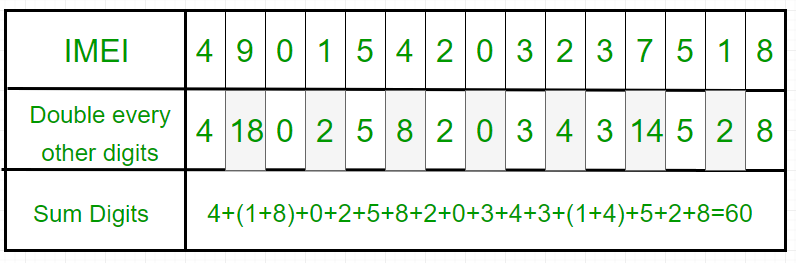# Program to check for a Valid IMEI Number

• Difficulty Level : Easy
• Last Updated : 28 Jul, 2022

International Mobile Equipment Identity (IMEI) is a number, usually unique, to identify mobile phones, as well as some satellite phones. It is usually found printed inside the battery compartment of the phone, but can also be displayed on-screen on most phones by entering *#06# on the dialpad, or alongside other system information in the settings menu on smartphone operating systems. The IMEI number is used by a GSM network to identify valid devices and therefore can be used for stopping a stolen phone from accessing that network.

The IMEI (15 decimal digits: 14 digits plus a check digit) includes information on the origin, model, and serial number of the device.

The IMEI is validated in following steps:

1. Starting from the rightmost digit, double the value of every second digit (e.g., 7 becomes 14).
2. If doubling of a number results in a two digits number i.e greater than 9(e.g., 7 × 2 = 14), then add the digits of the product (e.g., 14: 1 + 4 = 5), to get a single digit number.
3. Now take the sum of all the digits.
4. Check if the sum is divisible by 10 i.e.(total modulo 10 is equal to 0) then the IMEI number is valid; else it is not valid.

Example:

`Input IMEI : 490154203237518``Output : Since, 60 is divisible by 10, hence the given IMEI number is Valid.`

Implementation:

## C++

 `// C++ program to check whether the``// given EMEI number is valid or not.``#include``using` `namespace` `std;` `// Function for finding and returning``// sum of digits of a number``int` `sumDig(``int` `n)``{``    ``int` `a = 0;``    ``while` `(n > 0)``    ``{``        ``a = a + n % 10;``        ``n = n / 10;``    ``}``    ``return` `a;``}` `bool` `isValidIMEI(``long` `n)``{``    ` `    ``// Converting the number into ``    ``// String for finding length``    ``string s = to_string(n);``    ``int` `len = s.length();` `    ``if` `(len != 15)``        ``return` `false``;` `    ``int` `sum = 0;``    ``for``(``int` `i = len; i >= 1; i--)``    ``{``       ``int` `d = (``int``)(n % 10);``       ` `       ``// Doubling every alternate digit``       ``if` `(i % 2 == 0)``           ``d = 2 * d;``           ` `       ``// Finding sum of the digits``       ``sum += sumDig(d);``       ``n = n / 10;``    ``}``    ` `    ``return` `(sum % 10 == 0);``}` `// Driver code``int` `main()``{``    ``// 15 digits cannot be stored``    ``// in 'int' data type``    ``long` `n = 490154203237518L;` `    ``if` `(isValidIMEI(n))``        ``cout << ``"Valid IMEI Code"``;``    ``else``        ``cout << ``"Invalid IMEI Code"``;``    ` `    ``return` `0;``}` `// This code is contributed by Yash_R`

## Java

 `// Java program to check whether the``// given EMEI number is valid or not.``import` `java.io.*;``class` `IMEI``{``    ``// Function for finding and returning``    ``// sum of digits of a number``    ``static` `int` `sumDig(``int` `n)``    ``{``        ``int` `a = ``0``;``        ``while` `(n > ``0``)``        ``{``            ``a = a + n % ``10``;``            ``n = n / ``10``;``        ``}``        ``return` `a;``    ``}` `    ``static` `boolean` `isValidIMEI(``long` `n)``    ``{``        ``// Converting the number into String``        ``// for finding length``        ``String s = Long.toString(n);``        ``int` `len = s.length();` `        ``if` `(len != ``15``)``            ``return` `false``;` `        ``int` `sum = ``0``;``        ``for` `(``int` `i = len; i >= ``1``; i--)``        ``{``            ``int` `d = (``int``)(n % ``10``);` `            ``// Doubling every alternate digit``            ``if` `(i % ``2` `== ``0``)``                ``d = ``2` `* d;` `            ``// Finding sum of the digits``            ``sum += sumDig(d);``            ``n = n / ``10``;``        ``}` `        ``return` `(sum % ``10` `== ``0``);``    ``}` `    ``// Driver code``    ``public` `static` `void` `main(String args[]) ``throws` `IOException``    ``{``        ``// 15 digits cannot be stored in 'int' data type``        ``long` `n = 490154203237518L;` `        ``if` `(isValidIMEI(n))``            ``System.out.println(``"Valid IMEI Code"``);``        ``else``            ``System.out.println(``"Invalid IMEI Code"``);` `    ``}``}`

## Python3

 `# Python3 code to check whether the``# given EMEI number is valid or not` `# Function for finding and returning``# sum of digits of a number``def` `sumDig( n ):``    ``a ``=` `0``    ``while` `n > ``0``:``        ``a ``=` `a ``+` `n ``%` `10``        ``n ``=` `int``(n ``/` `10``)` `    ``return` `a` `# Returns True if n is valid EMEI``def` `isValidEMEI(n):` `    ``# Converting the number into``    ``# String for finding length``    ``s ``=` `str``(n)``    ``l ``=` `len``(s)` `    ``# If length is not 15 then IMEI is Invalid``    ``if` `l !``=` `15``:``        ``return` `False` `    ``d ``=` `0``    ``sum` `=` `0``    ``for` `i ``in` `range``(``15``, ``0``, ``-``1``):``        ``d ``=` `(``int``)(n ``%` `10``)``        ``if` `i ``%` `2` `=``=` `0``:` `            ``# Doubling every alternate digit``            ``d ``=` `2` `*` `d` `        ``# Finding sum of the digits``        ``sum` `=` `sum` `+` `sumDig(d)``        ``n ``=` `n ``/` `10``    ``return` `(``sum` `%` `10` `=``=` `0``)` `# Driver code``n ``=` `490154203237518``if` `isValidEMEI(n):``    ``print``(``"Valid IMEI Code"``)``else``:``    ``print``(``"Invalid IMEI Code"``)` `# This code is contributed by "Sharad_Bhardwaj".`

## C#

 `// C# program to check whether the``// given EMEI number is valid or not.``using` `System;` `class` `GFG {``    ` `    ``// Function for finding and``    ``// returning sum of digits``    ``// of a number``    ``static` `int` `sumDig(``int` `n)``    ``{``        ``int` `a = 0;``        ``while` `(n > 0)``        ``{``            ``a = a + n % 10;``            ``n = n / 10;``        ``}``        ` `        ``return` `a;``    ``}` `    ``static` `Boolean isValidIMEI(``long` `n)``    ``{``        ` `        ``// Converting the number into``        ``// String for finding length``        ``String s = n.ToString();``        ``int` `len = s.Length;` `        ``if` `(len != 15)``            ``return` `false``;` `        ``int` `sum = 0;``        ``for` `(``int` `i = len; i >= 1; i--)``        ``{``            ``int` `d = (``int``)(n % 10);` `            ``// Doubling every alternate``            ``// digit``            ``if` `(i % 2 == 0)``                ``d = 2 * d;` `            ``// Finding sum of the digits``            ``sum += sumDig(d);``            ``n = n / 10;``        ``}` `        ``return` `(sum % 10 == 0);``    ``}` `    ``// Driver code``    ``public` `static` `void` `Main()``    ``{` `        ``// 15 digits cannot be stored in``        ``// 'int' data type``        ``long` `n = 490154203237518L;` `        ``if` `(isValidIMEI(n))``            ``Console.Write(``"Valid IMEI Code"``);``        ``else``            ``Console.Write(``"Invalid IMEI Code"``);` `    ``}``}` `// This code is contributed by parashar.`

## Javascript

 ``

Output

`Valid IMEI Code`

Time complexity : O(n log10 n)
Auxiliary Space: O(n)

This article is contributed by Aarti_Rathi. If you like GeeksforGeeks and would like to contribute, you can also write an article using write.geeksforgeeks.org or mail your article to review-team@geeksforgeeks.org. See your article appearing on the GeeksforGeeks main page and help other Geeks.

My Personal Notes arrow_drop_up